## 5.6 Substitution with Definite Integrals

### Learning Objectives

• Use substitution to evaluate definite integrals.

# Substitution for Definite Integrals

Substitution can be used with definite integrals, too. However, using substitution to evaluate a definite integral requires a change to the limits of integration. If we change variables in the integrand, the limits of integration change as well.

### Substitution with Definite Integrals

Let $u=g(x)$ and let ${g}^{\text{′}}$ be continuous over an interval $\left[a,b\right],$ and let $f$ be continuous over the range of $u=g(x).$ Then,

${\int }_{a}^{b}f(g(x)){g}^{\prime }(x)dx={\int }_{g(a)}^{g(b)}f(u)du.$

Although we will not formally prove this theorem, we justify it with some calculations here. From the substitution rule for indefinite integrals, if $F(x)$ is an antiderivative of $f(x),$ we have

$\int f(g(x)){g}^{\prime }(x)dx=F(g(x))+C.$

Then

$\begin{array}{cc}{\int }_{a}^{b}f\left[g(x)\right]{g}^{\prime }(x)dx\hfill & ={F(g(x))|}_{x=a}^{x=b}\hfill \\ & =F(g(b))-F(g(a))\hfill \\ & ={F(u)|}_{u=g(a)}^{u=g(b)}\hfill \\ \\ \\ & ={\int }_{g(a)}^{g(b)}f(u)du,\hfill \end{array}$

and we have the desired result.

### Using Substitution to Evaluate a Definite Integral

Use substitution to evaluate ${\int }_{0}^{1}{x}^{2}{(1+2{x}^{3})}^{5}dx.$

Use substitution to evaluate the definite integral ${\int }_{-1}^{0}y{(2{y}^{2}-3)}^{5}dy.$

### Using Substitution with an Exponential Function

Use substitution to evaluate ${\int }_{0}^{1}x{e}^{4{x}^{2}+3}dx.$

Use substitution to evaluate ${\int }_{0}^{1}{x}^{2} \cos (\frac{\pi }{2}{x}^{3})dx.$

Substitution may be only one of the techniques needed to evaluate a definite integral. All of the properties and rules of integration apply independently, and trigonometric functions may need to be rewritten using a trigonometric identity before we can apply substitution. Also, we have the option of replacing the original expression for $u$ after we find the antiderivative, which means that we do not have to change the limits of integration. These two approaches are shown in (Figure).

### Using Substitution to Evaluate a Trigonometric Integral

Use substitution to evaluate ${\int }_{0}^{\pi \text{/}2}{ \cos }^{2}\theta d\theta .$

### Evaluating a Definite Integral Involving an Exponential Function

Evaluate the definite integral ${\int }_{1}^{2}{e}^{1-x}dx.$

Evaluate ${\int }_{0}^{2}{e}^{2x}dx.$

#### Hint

Let $u=2x.$

### Evaluating a Definite Integral Using Substitution

Evaluate the definite integral using substitution: ${\int }_{1}^{2}\frac{{e}^{1\text{/}x}}{{x}^{2}}dx.$

Evaluate the definite integral using substitution: ${\int }_{1}^{2}\frac{1}{{x}^{3}}{e}^{4{x}^{-2}}dx.$

#### Hint

Let $u=4{x}^{-2}.$

(Figure) is a definite integral of a trigonometric function. With trigonometric functions, we often have to apply a trigonometric property or an identity before we can move forward. Finding the right form of the integrand is usually the key to a smooth integration.

### Evaluating a Definite Integral

Find the definite integral of ${\int }_{0}^{\pi \text{/}2}\frac{ \sin x}{1+ \cos x}dx.$

### Evaluating a Definite Integral Using Inverse Trigonometric Functions

Evaluate the definite integral ${\int }_{0}^{1}\frac{dx}{\sqrt{1-{x}^{2}}}.$

### Evaluating a Definite Integral

Evaluate the definite integral ${\int }_{0}^{\sqrt{3}\text{/}2}\frac{du}{\sqrt{1-{u}^{2}}}.$

### Evaluating a Definite Integral

Evaluate the definite integral ${\int }_{\sqrt{3}\text{/}3}^{\sqrt{3}}\frac{dx}{1+{x}^{2}}.$

Evaluate the definite integral ${\int }_{0}^{2}\frac{dx}{4+{x}^{2}}.$

#### Hint

Follow the procedures from (Figure) to solve the problem.

In the following exercises, use a calculator to estimate the area under the curve using left Riemann sums with 50 terms, then use substitution to solve for the exact answer.

1. [T]$y=3{(1-x)}^{2}$ over $\left[0,2\right]$

2. [T]$y=x{(1-{x}^{2})}^{3}$ over $\left[-1,2\right]$

3. [T]$y= \sin x{(1- \cos x)}^{2}$ over $\left[0,\pi \right]$

4. [T]$y=\frac{x}{{({x}^{2}+1)}^{2}}$ over $\left[-1,1\right]$

In the following exercises, use a change of variables to evaluate the definite integral.

5. ${\int }_{0}^{1}x\sqrt{1-{x}^{2}}dx$

6. ${\int }_{0}^{1}\frac{x}{\sqrt{1+{x}^{2}}}dx$

7. ${\int }_{0}^{2}\frac{t}{\sqrt{5+{t}^{2}}}dt$

8. ${\int }_{0}^{1}\frac{t}{\sqrt{1+{t}^{3}}}dt$

9. ${\int }_{0}^{\pi \text{/}4}{ \sec }^{2}\theta \tan \theta d\theta$

10. ${\int }_{0}^{\pi \text{/}4}\frac{ \sin \theta }{{ \cos }^{4}\theta }d\theta$

In the following exercises, evaluate the indefinite integral $\int f(x)dx$ with constant $C=0$ using $u$-substitution. Then, graph the function and the antiderivative over the indicated interval. If possible, estimate a value of C that would need to be added to the antiderivative to make it equal to the definite integral $F(x)={\int }_{a}^{x}f(t)dt,$ with $a$ the left endpoint of the given interval.

11. [T]$\int (2x+1){e}^{{x}^{2}+x-6}dx$ over $\left[-3,2\right]$

12. [T]$\int \frac{ \cos (\text{ln}(2x))}{x}dx$ on $\left[0,2\right]$

13. [T]$\int \frac{3{x}^{2}+2x+1}{\sqrt{{x}^{3}+{x}^{2}+x+4}}dx$ over $\left[-1,2\right]$

14. [T]$\int \frac{ \sin x}{{ \cos }^{3}x}dx$ over $\left[-\frac{\pi }{3},\frac{\pi }{3}\right]$

15. [T]$\int (x+2){e}^{\text{−}{x}^{2}-4x+3}dx$ over $\left[-5,1\right]$

16. [T]$\int 3{x}^{2}\sqrt{2{x}^{3}+1}dx$ over $\left[0,1\right]$

17. If $h(a)=h(b)$ in ${\int }_{a}^{b}g\text{‘}(h(x))h(x)dx,$ what can you say about the value of the integral?

18. Is the substitution $u=1-{x}^{2}$ in the definite integral ${\int }_{0}^{2}\frac{x}{1-{x}^{2}}dx$ okay? If not, why not?

In the following exercises, use a change of variables to show that each definite integral is equal to zero.

19. ${\int }_{0}^{\pi }{ \cos }^{2}(2\theta ) \sin (2\theta )d\theta$

20. ${\int }_{0}^{\sqrt{\pi }}t \cos ({t}^{2}) \sin ({t}^{2})dt$

21. ${\int }_{0}^{1}(1-2t)dt$

22. ${\int }_{0}^{1}\frac{1-2t}{(1+{(t-\frac{1}{2})}^{2})}dt$

23. ${\int }_{0}^{\pi } \sin ({(t-\frac{\pi }{2})}^{3}) \cos (t-\frac{\pi }{2})dt$

24. ${\int }_{0}^{2}(1-t) \cos (\pi t)dt$

25. ${\int }_{\pi \text{/}4}^{3\pi \text{/}4}{ \sin }^{2}t \cos tdt$

26. Show that the average value of $f(x)$ over an interval $\left[a,b\right]$ is the same as the average value of $f(cx)$ over the interval $\left[\frac{a}{c},\frac{b}{c}\right]$ for $c>0.$

27. Find the area under the graph of $f(t)=\frac{t}{{(1+{t}^{2})}^{a}}$ between $t=0$ and $t=x$ where $a>0$ and $a\ne 1$ is fixed, and evaluate the limit as $x\to \infty .$

28. Find the area under the graph of $g(t)=\frac{t}{{(1-{t}^{2})}^{a}}$ between $t=0$ and $t=x,$ where $0<x<1$ and $a>0$ is fixed. Evaluate the limit as $x\to 1.$

29. The area of a semicircle of radius 1 can be expressed as ${\int }_{-1}^{1}\sqrt{1-{x}^{2}}dx.$ Use the substitution $x= \cos t$ to express the area of a semicircle as the integral of a trigonometric function. You do not need to compute the integral.

30. The area of the top half of an ellipse with a major axis that is the $x$-axis from $x=-1$ to $a$ and with a minor axis that is the $y$-axis from $y=\text{−}b$ to $b$ can be written as ${\int }_{\text{−}a}^{a}b\sqrt{1-\frac{{x}^{2}}{{a}^{2}}}dx.$ Use the substitution $x=a \cos t$ to express this area in terms of an integral of a trigonometric function. You do not need to compute the integral.

31. [T] The following graph is of a function of the form $f(t)=a \sin (nt)+b \sin (mt).$ Estimate the coefficients $a$ and $b$, and the frequency parameters $n$ and $m$. Use these estimates to approximate ${\int }_{0}^{\pi }f(t)dt.$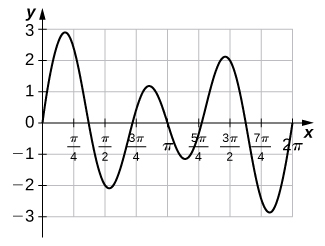32. [T] The following graph is of a function of the form $f(x)=a \cos (nt)+b \cos (mt).$ Estimate the coefficients $a$ and $b$ and the frequency parameters $n$ and $m$. Use these estimates to approximate ${\int }_{0}^{\pi }f(t)dt.$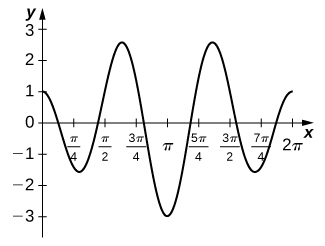33. ${\int }_{0}^{\pi \text{/}4} \tan xdx$

34. ${\int }_{0}^{\pi \text{/}3}\frac{ \sin x- \cos x}{ \sin x+ \cos x}dx$

35. ${\int }_{\pi \text{/}6}^{\pi \text{/}2} \csc xdx$

36. ${\int }_{\pi \text{/}4}^{\pi \text{/}3} \cot xdx$

In the following exercises, does the right-endpoint approximation overestimate or underestimate the exact area? Calculate the right endpoint estimate R50 and solve for the exact area.

37. [T]$y={e}^{x}$ over $\left[0,1\right]$

38. [T]$y={e}^{\text{−}x}$ over $\left[0,1\right]$

39. [T]$y=\text{ln}(x)$ over $\left[1,2\right]$

40. [T]$y=\frac{x+1}{{x}^{2}+2x+6}$ over $\left[0,1\right]$

41. [T]$y={2}^{x}$ over $\left[-1,0\right]$

42. [T]$y=\text{−}{2}^{\text{−}x}$ over $\left[0,1\right]$

In the following exercises, $f(x)\ge 0$ for $a\le x\le b.$ Find the area under the graph of $f(x)$ between the given values $a$ and $b$ by integrating.

43. $f(x)=\frac{{\text{log}}_{10}(x)}{x};a=10,b=100$

44. $f(x)=\frac{{\text{log}}_{2}(x)}{x};a=32,b=64$

45. $f(x)={2}^{\text{−}x};a=1,b=2$

46. $f(x)={2}^{\text{−}x};a=3,b=4$

47. Find the area under the graph of the function $f(x)=x{e}^{\text{−}{x}^{2}}$ between $x=0$ and $x=5.$

48. Compute the integral of $f(x)=x{e}^{\text{−}{x}^{2}}$ and find the smallest value of N such that the area under the graph $f(x)=x{e}^{\text{−}{x}^{2}}$ between $x=N$ and $x=N+10$ is, at most, 0.01.

49. Find the limit, as N tends to infinity, of the area under the graph of $f(x)=x{e}^{\text{−}{x}^{2}}$ between $x=0$ and $x=5.$

50. Show that ${\int }_{a}^{b}\frac{dt}{t}={\int }_{1\text{/}b}^{1\text{/}a}\frac{dt}{t}$ when $0<a\le b.$

51. Suppose that $f(x)>0$ for all $x$ and that $f$ and $g$ are differentiable. Use the identity ${f}^{g}={e}^{g\text{ln}f}$ and the chain rule to find the derivative of ${f}^{g}.$

52. Use the previous exercise to find the antiderivative of $h(x)={x}^{x}(1+\text{ln}x)$ and evaluate ${\int }_{2}^{3}{x}^{x}(1+\text{ln}x)dx.$

53. Show that if $c>0,$ then the integral of $1\text{/}x$ from ac to bc $(0<a<b)$ is the same as the integral of $1\text{/}x$ from $a$ to $b$.

The following exercises are intended to derive the fundamental properties of the natural log starting from the Definition/em> $\text{ln}(x)={\int }_{1}^{x}\frac{dt}{t},$ using properties of the definite integral and making no further assumptions.

54. Use the identity $\text{ln}(x)={\int }_{1}^{x}\frac{dt}{t}$ to derive the identity $\text{ln}(\frac{1}{x})=\text{−}\text{ln}x.$

55. Use a change of variable in the integral ${\int }_{1}^{xy}\frac{1}{t}dt$ to show that $\text{ln}xy=\text{ln}x+\text{ln}y\text{ for }x,y>0.$

56. Use the identity $\text{ln}x={\int }_{1}^{x}\frac{dt}{x}$ to show that $\text{ln}(x)$ is an increasing function of $x$ on $[0,\infty )$ and use the previous exercises to show that the range of $\text{ln}(x)$ is $(\text{−}\infty ,\infty ).$ Without any further assumptions, conclude that $\text{ln}(x)$ has an inverse function defined on $(\text{−}\infty ,\infty ).$

57. Pretend, for the moment, that we do not know that ${e}^{x}$ is the inverse function of $\text{ln}(x),$ but keep in mind that $\text{ln}(x)$ has an inverse function defined on $(\text{−}\infty ,\infty ).$ Call it E. Use the identity $\text{ln}xy=\text{ln}x+\text{ln}y$ to deduce that $E(a+b)=E(a)E(b)$ for any real numbers $a$, $b$.

58. Pretend, for the moment, that we do not know that ${e}^{x}$ is the inverse function of $\text{ln}x,$ but keep in mind that $\text{ln}x$ has an inverse function defined on $(\text{−}\infty ,\infty ).$ Call it E. Show that $E\text{‘}(t)=E(t).$

59. The sine integral, defined as $S(x)={\int }_{0}^{x}\frac{ \sin t}{t}dt$ is an important quantity in engineering. Although it does not have a simple closed formula, it is possible to estimate its behavior for large $x$. Show that for $k\ge 1,|S(2\pi k)-S(2\pi (k+1))|\le \frac{1}{k(2k+1)\pi }.$(Hint: $\sin (t+\pi )=\text{−} \sin t$)

60. [T] The normal distribution in probability is given by $p(x)=\frac{1}{\sigma \sqrt{2\pi }}{e}^{\text{−}{(x-\mu )}^{2}\text{/}2{\sigma }^{2}},$ where σ is the standard deviation and μ is the average. The standard normal distribution in probability, ${p}_{s},$ corresponds to $\mu =0\text{ and }\sigma =1.$ Compute the left endpoint estimates ${R}_{10}\text{ and }{R}_{100}$ of ${\int }_{-1}^{1}\frac{1}{\sqrt{2\pi }}{e}^{\text{−}{x}^{2\text{/}2}}dx.$

61. [T] Compute the right endpoint estimates ${R}_{50}\text{ and }{R}_{100}$ of ${\int }_{-3}^{5}\frac{1}{2\sqrt{2\pi }}{e}^{\text{−}{(x-1)}^{2}\text{/}8}.$

In the following exercises, evaluate each integral in terms of an inverse trigonometric function.

62. ${\int }_{0}^{\sqrt{3}\text{/}2}\frac{dx}{\sqrt{1-{x}^{2}}}$

63. ${\int }_{-1\text{/}2}^{1\text{/}2}\frac{dx}{\sqrt{1-{x}^{2}}}$

64. ${\int }_{\sqrt{3}}^{1}\frac{dx}{\sqrt{1+{x}^{2}}}$

65. ${\int }_{1\text{/}\sqrt{3}}^{\sqrt{3}}\frac{dx}{1+{x}^{2}}$

66. ${\int }_{1}^{\sqrt{2}}\frac{dx}{|x|\sqrt{{x}^{2}-1}}$

67. ${\int }_{1}^{2\text{/}\sqrt{3}}\frac{dx}{|x|\sqrt{{x}^{2}-1}}$

68. Explain what is wrong with the following integral: ${\int }_{1}^{2}\frac{dt}{\sqrt{1-{t}^{2}}}.$

69. Explain what is wrong with the following integral: ${\int }_{-1}^{1}\frac{dt}{|t|\sqrt{{t}^{2}-1}}.$

In the following exercises, solve for the antiderivative $\int f$ of $f$ with $C=0,$ then use a calculator to graph $f$ and the antiderivative over the given interval $\left[a,b\right].$ Identify a value of C such that adding C to the antiderivative recovers the definite integral $F(x)={\int }_{a}^{x}f(t)dt.$

70. [T]$\int \frac{1}{\sqrt{9-{x}^{2}}}dx$ over $\left[-3,3\right]$

71. [T]$\int \frac{9}{9+{x}^{2}}dx$ over $\left[-6,6\right]$

72. [T]$\int \frac{ \cos x}{4+{ \sin }^{2}x}dx$ over $\left[-6,6\right]$

73. [T]$\int \frac{{e}^{x}}{1+{e}^{2x}}dx$ over $\left[-6,6\right]$

In the following exercises, use a calculator to graph the antiderivative $\int f$ with $C=0$ over the given interval $\left[a,b\right].$ Approximate a value of C, if possible, such that adding C to the antiderivative gives the same value as the definite integral $F(x)={\int }_{a}^{x}f(t)dt.$

74. [T]$\int \frac{1}{x\sqrt{{x}^{2}-4}}dx$ over $\left[2,6\right]$

75. [T]$\int \frac{1}{(2x+2)\sqrt{x}}dx$ over $\left[0,6\right]$

76. [T]$\int \frac{( \sin x+x \cos x)}{1+{x}^{2}{ \sin }^{2}x}dx$ over $\left[-6,6\right]$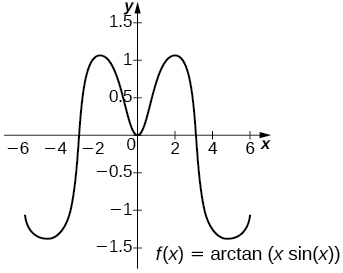The general antiderivative is ${ \tan }^{-1}(x \sin x)+C.$ Taking $C=\text{−}{ \tan }^{-1}(6 \sin (6))$ recovers the definite integral.

77. [T]$\int \frac{2{e}^{-2x}}{\sqrt{1-{e}^{-4x}}}dx$ over $\left[0,2\right]$

78. [T]$\int \frac{1}{x+x{\text{ln}}^{2}x}$ over $\left[0,2\right]$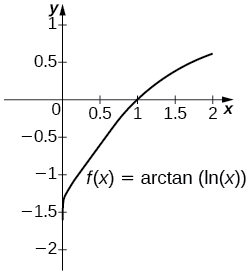The general antiderivative is ${ \tan }^{-1}(\text{ln}x)+C.$ Taking $C=\frac{\pi }{2}={ \tan }^{-1}\infty$ recovers the definite integral.

79. [T]$\int \frac{{ \sin }^{-1}x}{\sqrt{1-{x}^{2}}}$ over $\left[-1,1\right]$

In the following exercises, compute each definite integral.

80. ${\int }_{0}^{1\text{/}2}\frac{ \tan ({ \sin }^{-1}t)}{\sqrt{1-{t}^{2}}}dt$

81. ${\int }_{1\text{/}4}^{1\text{/}2}\frac{ \tan ({ \cos }^{-1}t)}{\sqrt{1-{t}^{2}}}dt$

82. ${\int }_{0}^{1\text{/}2}\frac{ \sin ({ \tan }^{-1}t)}{1+{t}^{2}}dt$

83. ${\int }_{0}^{1\text{/}2}\frac{ \cos ({ \tan }^{-1}t)}{1+{t}^{2}}dt$

84. For $A>0,$ compute $I(A)={\int }_{\text{−}A}^{A}\frac{dt}{1+{t}^{2}}$ and evaluate $\underset{a\to \infty }{\text{lim}}I(A),$ the area under the graph of $\frac{1}{1+{t}^{2}}$ on $\left[\text{−}\infty ,\infty \right].$

85. Use the following graph to prove that ${\int }_{0}^{x}\sqrt{1-{t}^{2}}dt=\frac{1}{2}x\sqrt{1-{x}^{2}}+\frac{1}{2}\phantom{\rule{0.05em}{0ex}}{ \sin }^{-1}x.$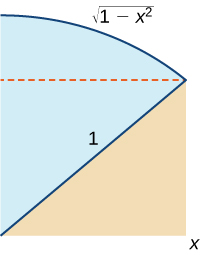## Glossary

change of variables
the substitution of a variable, such as $u$, for an expression in the integrand
integration by substitution
a technique for integration that allows integration of functions that are the result of a chain-rule derivative